## 传统方法

c++11之前一般会用`srand()`配合`rand()`来实现随机数的使用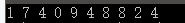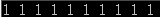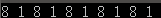## c++11随机数算法

c++11提供了3个新的随机算法

• linear_congruential_engine线性同余法
• mersenne_twister_engine梅森旋转法
• substract_with_carry_engine滞后Fibonacci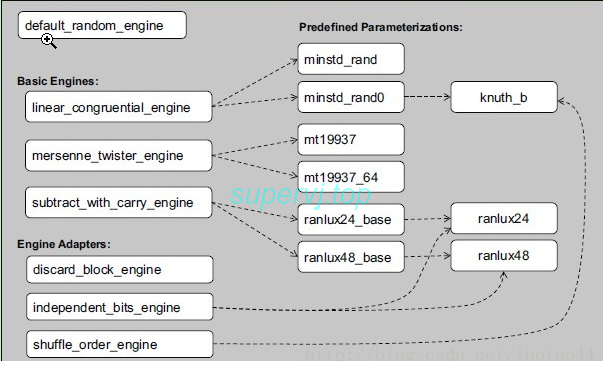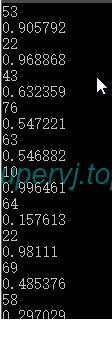uniform_int_distribution的随机数的范围是 `[ ]`

uniform_real_distribution却是半开范围 ` [ )`

## 其他算法

C++11提供的概率分布类型有下面这些：

``````uniform_int_distribution          整数均匀分布
uniform_real_distribution        浮点数均匀分布``````

``````bernoulli_distribution    伯努利分布
binomial_distribution     二项分布
geometry_distribution    几何分布
negative_biomial_distribution  负二项分布``````

Rate-based distributions:

``````poisson_distribution 泊松分布
exponential_distribution指数分布
gamma_distribution 伽马分布
weibull_distribution 威布尔分布
extreme_value_distribution 极值分布``````

``````normal_distribution        正态分布
chi_squared_distribution卡方分布
cauchy_distribution       柯西分布
fisher_f_distribution      费歇尔F分布
student_t_distribution t分布``````

``````discrete_distribution离散分布
piecewise_constant_distribution分段常数分布
piecewise_linear_distribution分段线性分布``````

### 泊松分布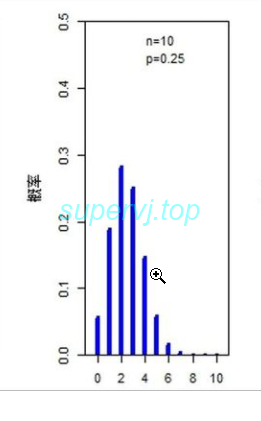微信支付宝